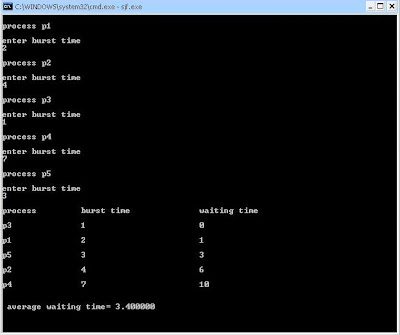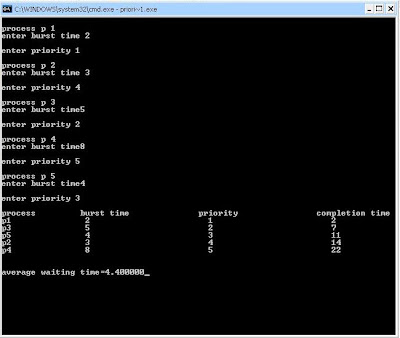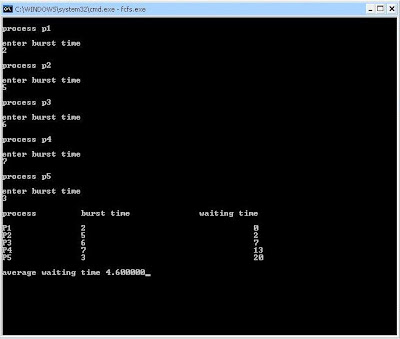### Destroy a session in PHP

here is the script for destroying a session.......

< ?php
session_start();
session_destroy();
?>

### ATM - An alarm to police

If you are ever forced by a thief or someone to take money out of an ATM
Machine; enter your pin number reversed.
So if your number is 1254 mark 4521.

The ATM machine will give you your money, but will automatically recognize this as a plea for help and will alert the police unknown to the thief.

This option is in all ATM machines, but not many people know this.

Please pass this information on to others. No harm in keeping this in mind!!

### IIT Roorkee Cognizance-2009

I was at iit roorkee when i make this video of two IITian a singer and a guitarist...

that was amazing moment

and that night is unforgettable to me.
here is that video...

### Shortest Job First (SJF)-CPU Scheduling Algo in C

simulation of CPU Scheduling Algorithm - SJF in C

Code goes like that

# include < stdio.h>
# include < conio.h>

struct process
{
int pid ;
int burst ;
int wait ;
};
void main()
{
struct process p , temp ;
int i,j,wait=0 ;
float twait;
clrscr();
for(i=0;i<5;i++)
{
p[i].pid = i+1 ;
printf("\nprocess p%d\n",i+1) ;
printf("\nenter burst time\n");
scanf("%d",&p[i].burst);
}
for(i=0;i<5;i++)
{ for(j=0;j<5;j++)
{
if(p[j].burst>p[i].burst)
{
temp=p[i];
p[i]=p[j] ;
p[j]=temp;
}
}
}
printf("\nprocess\t\tburst time\t\twaiting time\n");
for(i=0;i<5;i++)
{
p[i].wait=wait ;
printf("\np%d\t\t%d\t\t\t%d\n",p[i].pid,p[i].burst,p[i].wait);
wait = wait + p[i].burst ;
}
twait=wait;
printf("\n\n average waiting time= %f",(twait/5));
getch();
}### Priority Scheduling in C

Simulation of CPU Scheduling Algorithm - Priority Scheduling in C

code goes like that

#include< stdio.h>
#include< conio.h>
struct process
{
int pid;
int priority;
int comp;
int burst;
};
void main()
{
struct process p, temp;
int i,j, comp=0;
float tcomp;
clrscr();
for(i=0;i<5;i++)
{
p[i].pid = i+1;
printf("\nprocess p %d",i+1);
printf("\nenter burst time");
scanf("\n%d",&p[i].burst);
printf("\nenter priority ");
scanf("\n%d",&p[i].priority);
}
for(i=0;i<5;i++)
{
for(j=0;j<5;j++)
{
if(p[j].priority>p[i].priority)
{ temp=p[i];
p[i]=p[j];
p[j]=temp;
}
}
}
printf("\nprocess\t\tburst time\t\tpriority\t\tcompletion time\n");
for(i=0;i<5;i++)
{
{
comp=comp+p[i].burst;
}
printf("p%d\t\t %d\t\t\t %d\t\t\t %d\n",p[i].pid,p[i].burst,p[i].priority,comp);
}
tcomp=comp;
printf("\n\naverage waiting time=%f",(tcomp/5));
getch();
}

output for the above code is:### FCFS-CPU Scheduling in C

i was searching for source code for simulation of CPU Scheduling Algorithm FCFS in C on google
so i am going to post it here!

#include < stdio.h>
#include < conio.h>
struct process
{
int pid;
int burst;
int wait;
};
void main()
{
struct process p;
int i, j, wait=0;
float twait;
clrscr();
for(i=1;i<=5;i++)
{
p[i].pid=i;
printf("\nprocess p%d\n",i);
printf("\nenter burst time\n");
scanf("%d",&p[i].burst);
}
printf("\nprocess\t\tburst time\t\twaiting time\n");
for(i=1;i<=5;i++)
{
p[i].pid=i;
p[i].wait=wait;
printf("\nP%d\t\t%d\t\t\t\t %d",p[i].pid, p[i].burst,p[i].wait);
wait = wait + p[i].burst;
}
twait=wait;
printf("\n\naverage waiting time= %f",(twait/5));
getch();
}

Output for this code looks like this